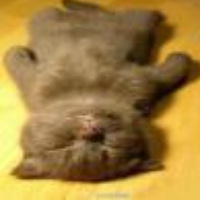# 翻译:计算机视觉中的边缘检测

## 边缘检测：高斯滤波器

`高斯滤波器`是边缘检测最基础的滤波器之一，虽然还有其他的，但是`高斯滤波器`会贯穿这篇文章。`高斯滤波器`，正如其名，是一个基于高斯分布的滤波器。

• 我的猫 `Mew` 的图片

• 对我的猫 `Mew` 的图片应用`高斯滤波`

``````% Takes the derivative of a 5x5 gaussian, with a sigma
[hx, hy] = gradient(fspecial('gaussian',[5 5],sigma));
``````

## 边缘检测：应用滤波器

``````% Convert an image to double for increased precision
img = double(img);
% Find two derived gaussians with respect to x and y
[hx, hy] = gradient(fspecial('gaussian',[5 5],sigma));
% Run the filters over the image, generating a filtered image
% Leaves x edges
gx = double(imfilter(img,hx,'replicate', 'conv'));
% Leaves y edges
gy = double(imfilter(img,hy,'replicate', 'conv'));
% Take the absolute value, and combine the x and y edges
mag = sqrt((gx .* gx) + (gy .* gy));
% Use non-maxima suppression
[mag, ] = max(mag, [], 3);
``````

• 基本边缘滤波器: 对我的猫的黑白图片应用滤波器

• 基本边缘滤波器: 对我的猫的`RGB`图片应用滤波器

## 边缘检测：方向滤波器

``````% Create four filters
[hx, hy] = gradient(fspecial('gaussian',[5 5],sigma));
[hx1, hy1] = altOrientFilter1(hx, hy);
[hx2, hy2] = altOrientFilter2(hx, hy);
[hx3, hy3] = altOrientFilter3(hx, hy);

% Run first gaussian filter on image
gx = double(imfilter(img,hx,'replicate', 'conv'));
gy = double(imfilter(img,hy,'replicate', 'conv'));
% Run second gaussian filter on image
gx1 = double(imfilter(img,hx1,'replicate', 'conv'));
gy1 = double(imfilter(img,hy1,'replicate', 'conv'));
% Run third gaussian filter on image
gx2 = double(imfilter(img,hx2,'replicate', 'conv'));
gy2 = double(imfilter(img,hy2,'replicate', 'conv'));
% Run fourth gaussian filter on image
gx3 = double(imfilter(img,hx3,'replicate', 'conv'));
gy3 = double(imfilter(img,hy3,'replicate', 'conv'));

% Merge all filters
squareGD = (gx .* gx) + (gy .* gy);
squareGD = squareGD + (gx1 .* gx1) + (gy1 .* gy1);
squareGD = squareGD + (gx2 .* gx2) + (gy2 .* gy2);
squareGD = squareGD + (gx3 .* gx3) + (gy3 .* gy3);

% Run non-maxima supression
[mag, ] = max(sqrt(squareGD), [], 3);
``````

## 边缘检测：提高彩色域

`Lab` 彩色空间的每个字母表示：

• `L` —— Luminance 亮度
• `a` —— alpha —— 红到绿
• `b` —— beta —— 黄到蓝

``````% Convert an image to the Lab color space
colorTransform = makecform('srgb2lab');
img = applycform(rgbImg, colorTransform);

% Make it double to improve representation
img = double(img);

% Find x and y derivative of a 9x9 gaussian
[hx, hy] = gradient(fspecial('gaussian',[9 9],sigma));

% Apply filters
gx = double(imfilter(img,hx,'replicate'));
gy = double(imfilter(img,hy,'replicate'));

% Find absolute value
gSquared = sqrt(gx .* gx) + (gy .* gy);

% Apply non-maxima suppression (find best points for edges)
[mag, ] = max(gSquared, [], 3);
``````

• Windows XP 背景的Lab空间图像

• `Lab 彩色域`上边缘检测

• `Lab 彩色域`边缘检测

## 参考文献

Canny, John. “A computational approach to edge detection.” Pattern Analysis and Machine Intelligence, IEEE Transactions on 6 (1986): 679-698.

Freeman, William T., and Edward H. Adelson. “The design and use of steerable filters.” IEEE Transactions on Pattern analysis and machine intelligence 13.9 (1991): 891-906.### FreeBlues

Terminator2050
2018/08/27
0
0

【方向】
2018/03/15
0
0

2018/08/16
0
0

zchang81
2018/01/17
0
0
opencv动态边缘检测-python

OpenCV：是一个基于BSD许可（开源）发行的跨平台计算视觉库。由一系列C函数和少量的C++编写，实现图像处理和计算机视觉方面的很多通用算法。 Canny边缘检测算子：是一种多级检测算法。1986年...

yan_shichang
2018/04/24
0
0

Java单例模式学习记录

JerryLin123

1
0
VSCODE 无法调试

VSCODE 无法调试 可以运行 可能的原因： GCC 的参数忘了加 -g

shzwork

3
0

Tiny熊

4
0
5.线程实现

Eappo_Geng

3
0

Cobbage

5
0